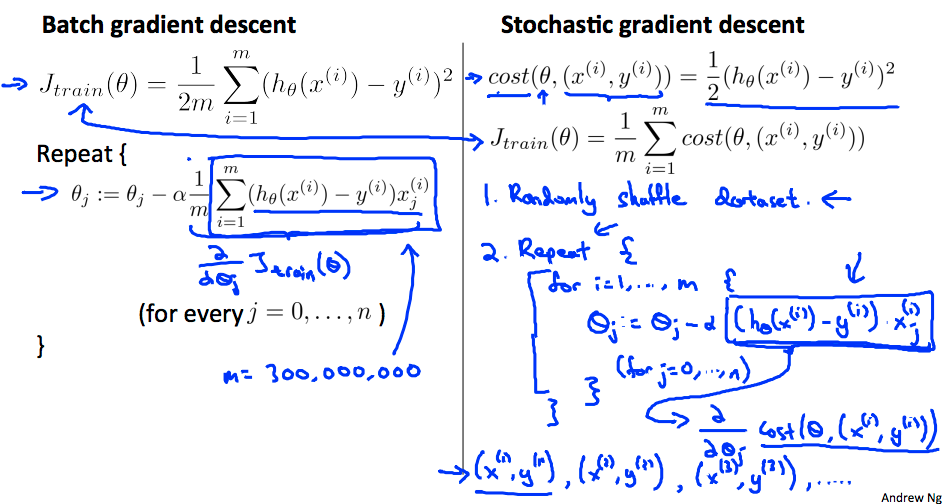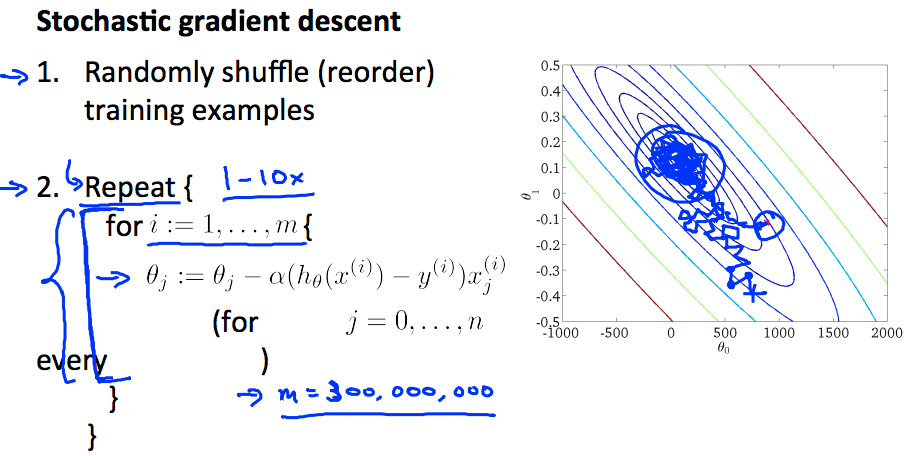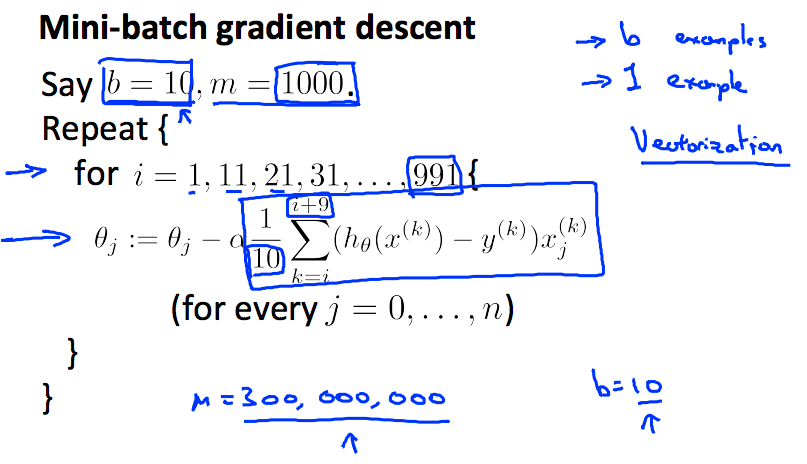# Learning With Large Datasets

$hθ (x) = ∑ j = 0 n θj xj$

$J ( θ ) = 1 2 m ∑ i = 1 m h θ ( x (i) ) − y (i) 2$

$θ j := θ j − α 1 m ∑ i = 1 m ( h θ ( x ( i ) ) − y ( i ) ) ⋅ x j ( i )$• Repeat 的次数可以选择 1-10，当样本量足够大时，一次内循环就够了
• 随机梯度下降可以保证参数朝着全局最小值的方向被更新，但无法保证下降的顺序（收敛形式不同）和最终的梯度值不发生变化
• 随机梯度下降是在靠近全局最小值的区域内徘徊，而不是直接得到全局最小值并停留在那个点上
• Learning Rate α 的值通常为常量，如果希望 θ 完全收敛，可以让 α 随时间减小( $\alpha =\frac{\text{const1}}{\text{iterationNumber}+\text{const2}}$)，通常来说不需要这么做